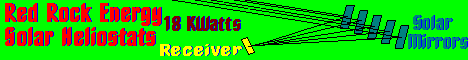°
Analemma
The solar tracking software Project.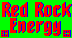Go back to Red Rock Energy.
45.08096°N
45° 4' 51.456"N
-93.02377°W
-93° 1' 25.572"W
Analemma  Books  Digital Sundial  Links  Moshier  Paul Schlyter  Solar Pathfinder

analemmaThe Analemma is the key to astronomical and solar tracking mathematics.How to calculate alt/az of sun for any time/location - Do It Yourself.
Accurate to about 1/10th of a degree.

digi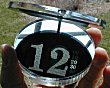A digital sundialRichard D. Swensen's Sundial at the University of Wisconsin River Falls.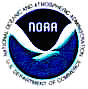NOAA Surface Radiation Research Branch Solar Position Calculator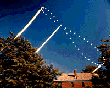The equation of time.Definitions of Astronomical Terms.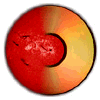Solar Ephemeris Calculations.Dan's Astronomy Software.solar.bas By Michael A. Syczylo

checksuncheckSun.
Spectrum
Galileo/GPS controlled Solar Tracking
Freeware/GPL context

susdesign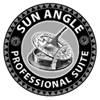Sustainable By Design
provides a suite of shareware design tools on sustainable energy topics including solar position and path diagrams.

blackberryHelioStat for BlackBerry Smartphones
Find sunrise, sunset, moonrise, moonset and the location of the sun for any time and date.
This program runs in a Blackberry and can, among other info, display the current AZimuth and ALTitude of the sun.
\$6.99us

pathfinder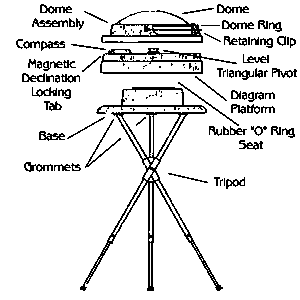The Solar Pathfinder.

solardatSun chart program. Generates sun path diagrams in PDF format.

nrel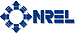Solar Position Algorithm for Solar Radiation Applications
NREL has published this document to help designers of solar concentrator develop software to track the sun in high resolution.

iesmithSolar Calculator 2.0
The Solar Calculator can be a very useful tool for a photographer. It gives an easy to read graphical indication of the position of the sun and moon at both rise and set, as well as their current positions in the sky, and it can do this for just about any location on any time and date you wish.

books
Books.

I have arranged the order of these books with the most desirable at the top.

by Peter Duffett-Smith
Edition Desc: 2nd ed
Published 1990
ISBN 0 521 38093 6 hardback \$74.95
ISBN 0 521 38995 X Paperback \$30.95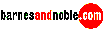Get the out of print book here.
The software in this book is based written in Microsoft's GW Basic. The equations are very extensive.
BTW you want the second edition because it has more routines than the 1st.
I would recommend obtaining a copy of this book before all others. His method of presenting the equations and the resultant programs is masterful. One can take his programs and easily modify them for special uses. This book is required reading for those who are writing astronomical software. Even if you are writing low accuracy algorithms, such as for solar tracking, his programs will give you a benchmark to compare the results.Download the software from here.
This is a self extracting compressed file. Put it in a separate directory and run it.

Astronomical Formulae for Calculators
by Jean H. Meeus
Edition Desc: 4th ed
Published 1988
ISBN 0 943 39622 0 Paperback \$22.75Get the out of print book here.
If I had no other books, this is the one to have. Meeus is recognized as the authority on astronomical calculations. Others always refer to him for reference.

by Peter Duffett-Smith
Edition Desc: 3rd ed
Published 1988
ISBN 0 521 35629 6 hardback \$54.95usGet the out of print book here.
The third edition of a guide book for amateur astronomers features new sections on generalized coordinate transformations, nutations, aberration, and selenographic coordinates as well as improvements to the sunrise and moonrise calculations.

Easy PC Astronomy
by Peter Duffett-Smith
Edition Desc: book & disk
Published 1996
ISBN 0 521 56052 7 Paperback \$30.95Get the book here.
Easy PC Astronomy is the book for all those who want to make astronomical calculations easily and accurately. A simple but powerful script language called AstroScript is provided on a disk with the book, ready to use on any IBM PC-type computer.
I don't have this book myself, but it sounds good.

heavensaboveWelcome to Heavens-Above.
If you're interested in satellites or astronomy, you've come to the right place! Our aim is to provide you with all the information you need to observe satellites such as the International Space Station and the Space Shuttle, spectacular events such as the dazzlingly bright flares from Iridium satellites as well as a wealth of other spaceflight and astronomical information.

bartels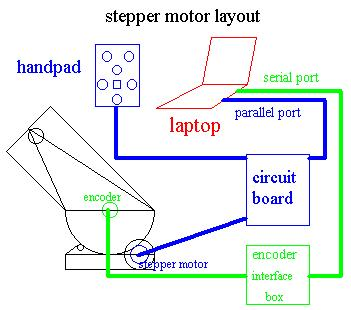Computer Operated Telescopes
By Mel Bartels

solpos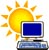Solar Position and Intensity
NREL's SOLPOS 2.0

Source code written in "C"

axel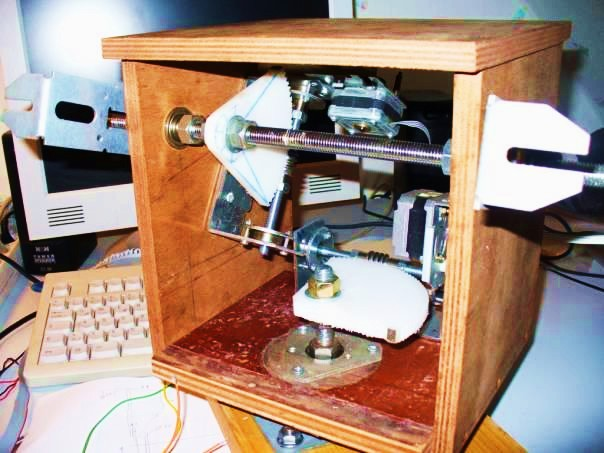* Axel's Helioworm Heliostat.
Helioworm - DIY Heliostat drive with self made worm gear.
The worm gear wheel is made from the white plastic of a cutting board.
The stepper motors and the gears are taken from an old printer.
All moving parts and the control electronics are located in the housing and are the weather proof.
The control unit is based on an Atmel Mega168 micro controller.
Electronic board and control program.

mowciusthe ramblings of mowcius
sun tracker code

mowcius has written a program to drive a heliostat using stepper motors. Source code written in "C" for the Pololu Arduino.

cerebralCerebral Meltdown
Arduino Sun Tracking / Heliostat Electronics Control System

This guy has written a program to drive a heliostat using stepper motors. Source code written in "C" for the Pololu Arduino.

psaPSA Algorithm Files
Arduino Sun Tracking / Heliostat Electronics Control System

Spanish open source sun position code for a heliostat. Source code written in "C++" for the Pololu Arduino.
Happylab wiki.

```// SunPos.h
// This file is available in electronic form at http://www.psa.es/sdg/sunpos.htm

#ifndef __SUNPOS_H
#define __SUNPOS_H

// Declaration of some constants
#define pi    3.14159265358979323846
#define twopi (2*pi)
#define dEarthMeanRadius     6371.01	// In km
#define dAstronomicalUnit    149597890	// In km

struct cTime
{
int iYear;
int iMonth;
int iDay;
double dHours;
double dMinutes;
double dSeconds;
};

struct cLocation
{
double dLongitude;
double dLatitude;
};

struct cSunCoordinates
{
double dZenithAngle;
double dAzimuth;
};

void sunpos(cTime udtTime, cLocation udtLocation, cSunCoordinates *udtSunCoordinates);

#endif```
```// SunPos.cpp
// This file is available in electronic form at http://www.psa.es/sdg/sunpos.htm

#include "sunpos.h"
#include

void sunpos(cTime udtTime,cLocation udtLocation, cSunCoordinates *udtSunCoordinates)
{
// Main variables
double dElapsedJulianDays;
double dDecimalHours;
double dEclipticLongitude;
double dEclipticObliquity;
double dRightAscension;
double dDeclination;

// Auxiliary variables
double dY;
double dX;

// Calculate difference in days between the current Julian Day
// and JD 2451545.0, which is noon 1 January 2000 Universal Time
{
double dJulianDate;
long int liAux1;
long int liAux2;
// Calculate time of the day in UT decimal hours
dDecimalHours = udtTime.dHours + (udtTime.dMinutes
+ udtTime.dSeconds / 60.0 ) / 60.0;
// Calculate current Julian Day
liAux1 =(udtTime.iMonth-14)/12;
liAux2=(1461*(udtTime.iYear + 4800 + liAux1))/4 + (367*(udtTime.iMonth
- 2-12*liAux1))/12- (3*((udtTime.iYear + 4900
+ liAux1)/100))/4+udtTime.iDay-32075;
dJulianDate=(double)(liAux2)-0.5+dDecimalHours/24.0;
// Calculate difference between current Julian Day and JD 2451545.0
dElapsedJulianDays = dJulianDate-2451545.0;
}

// Calculate ecliptic coordinates (ecliptic longitude and obliquity of the
// ecliptic in radians but without limiting the angle to be less than 2*Pi
// (i.e., the result may be greater than 2*Pi)
{
double dMeanLongitude;
double dMeanAnomaly;
double dOmega;
dOmega=2.1429-0.0010394594*dElapsedJulianDays;
dMeanLongitude = 4.8950630+ 0.017202791698*dElapsedJulianDays; // Radians
dMeanAnomaly = 6.2400600+ 0.0172019699*dElapsedJulianDays;
dEclipticLongitude = dMeanLongitude + 0.03341607*sin( dMeanAnomaly )
+ 0.00034894*sin( 2*dMeanAnomaly )-0.0001134
-0.0000203*sin(dOmega);
dEclipticObliquity = 0.4090928 - 6.2140e-9*dElapsedJulianDays
+0.0000396*cos(dOmega);
}

// Calculate celestial coordinates ( right ascension and declination ) in radians
// but without limiting the angle to be less than 2*Pi (i.e., the result may be
// greater than 2*Pi)
{
double dSin_EclipticLongitude;
dSin_EclipticLongitude= sin( dEclipticLongitude );
dY = cos( dEclipticObliquity ) * dSin_EclipticLongitude;
dX = cos( dEclipticLongitude );
dRightAscension = atan2( dY,dX );
if( dRightAscension < 0.0 ) dRightAscension = dRightAscension + twopi;
dDeclination = asin( sin( dEclipticObliquity )*dSin_EclipticLongitude );
}

// Calculate local coordinates ( azimuth and zenith angle ) in degrees
{
double dGreenwichMeanSiderealTime;
double dLocalMeanSiderealTime;
double dHourAngle;
double dCos_Latitude;
double dSin_Latitude;
double dCos_HourAngle;
double dParallax;
dGreenwichMeanSiderealTime = 6.6974243242 +
0.0657098283*dElapsedJulianDays
+ dDecimalHours;
dLocalMeanSiderealTime = (dGreenwichMeanSiderealTime*15
dHourAngle = dLocalMeanSiderealTime - dRightAscension;
dCos_HourAngle= cos( dHourAngle );
udtSunCoordinates->dZenithAngle = (acos( dCos_Latitude*dCos_HourAngle
*cos(dDeclination) + sin( dDeclination )*dSin_Latitude));
dY = -sin( dHourAngle );
dX = tan( dDeclination )*dCos_Latitude - dSin_Latitude*dCos_HourAngle;
udtSunCoordinates->dAzimuth = atan2( dY, dX );
if ( udtSunCoordinates->dAzimuth < 0.0 )
udtSunCoordinates->dAzimuth = udtSunCoordinates->dAzimuth + twopi;
// Parallax Correction
*sin(udtSunCoordinates->dZenithAngle);
udtSunCoordinates->dZenithAngle=(udtSunCoordinates->dZenithAngle
}
}```

burnettPosition of the Sun
By Keith Burnett
Accurate to about 1/40th of a degree.
OK, this site no longer exists. http://www.stargazing.net/kepler/sun.html

```'*********************************************************
'   This program will calculate the position of the Sun
'   using a low precision method found on page C24 of the
'   1996 Astronomical Almanac.
'
'   The method is good to 0.01 degrees in the sky over the
'   period 1950 to 2050.
'
'   QBASIC program by Keith Burnett  (http://bodmas.org/kepler/sun.html)
'
'   Work in double precision and define some constants
'
DEFDBL A-Z
pr1\$ = "\         \#####.##"
pr2\$ = "\         \#####.#####"
pr3\$ = "\         \#####.###"
pi = 4 * ATN(1)
tpi = 2 * pi
twopi = tpi
degs = 180 / pi
'
'   Get the days to J2000
'   h is UT in decimal hours
'   FNday only works between 1901 to 2099 - see Meeus chapter 7
'
DEF FNday (y,m,d,h) = 367 * y - 7 * (y + (m + 9) \ 12) \ 4 + 275 * m\ 9 + d - 730531.5 + h / 24
'
'   define some arc cos and arc sin functions and
'   a modified inverse tangent function
'
DEF FNacos (x)
s = SQR(1 - x * x)
FNacos = ATN(s / x)
END DEF
DEF FNasin (x)
c = SQR(1 - x * x)
FNasin = ATN(x / c)
END DEF
'
'   the atn2 function below returns an angle in the
'   range 0 to two pidepending on the signs of x and y.
'
DEF FNatn2 (y, x)
a = ATN(y / x)
IF x < 0 THEN a = a + pi
IF (y < 0) AND (x > 0) THEN a = a + tpi
FNatn2 = a
END DEF
'
'   the function below returns the true integer part,
'   even for negative numbers
'
DEF FNipart (x) = SGN(x) * INT(ABS(x))
'
'   the function below returns an angle in the range
'   0 to two pi
'
DEF FNrange (x)
b = x / tpi
a = tpi * (b - FNipart(b))
IF a < 0 THEN a = tpi + a
FNrange = a
END DEF
'
'   Find the ecliptic longitude of the Sun
'
DEF FNsun (d)
'
'   mean longitude of the Sun
'
'
'   mean anomaly of the Sun
'
'
'   Ecliptic longitude of the Sun
'
FNsun = FNrange(L + 1.915 * rads * SIN(g) + .02 * rads * SIN(2 * g))
'
'   Ecliptic latitude is assumed to be zero by definition
'
END DEF
'
'
'
CLS
'
'    get the date and time from the user
'
' INPUT "  year  : ", y
' INPUT "  month : ", m
' INPUT "  day   : ", day
' INPUT "hour UT : ", h
' INPUT " minute : ", mins
' INPUT "    lat : ", glat
' INPUT "   long : ", glong

y = 2002
m = 7
day = 9
h = 0
mins = 23
glat = 45.08096
glong = -93.02377

h = h + mins / 60
d = FNday(y, m, day, h)
'
'   Use FNsun to find the ecliptic longitude of the
'   Sun
'
lambda = FNsun(d)
'
'   Obliquity of the ecliptic
'
'
'   Find the RA and DEC of the Sun
'
alpha = FNatn2(COS(obliq) * SIN(lambda), COS(lambda))
delta = FNasin(SIN(obliq) * SIN(lambda))
'
'   Find the Earth - Sun distance
'
r = 1.00014 - .01671 * COS(g) - .00014 * COS(2 * g)
'
'   Find the Equation of Time
'
equation = (L - alpha) * degs * 4
'
'   find the Alt and Az of the Sun for a given position
'   on Earth
'
'   hour angle of Sun
LMST = FNrange((280.46061837# + 360.98564736629# * d) * rads + glong)
hasun = FNrange(LMST - alpha)
'
'   conversion from hour angle and dec to Alt Az
sinalt = SIN(delta) * SIN(glat) + COS(delta) * COS(glat) * COS(hasun)
altsun = FNasin(sinalt)
' y = -COS(delta) * COS(glat) * SIN(hasun)
alt = -COS(delta) * COS(glat) * SIN(hasun)
' x = SIN(delta) - SIN(glat) * sinalt
az  = SIN(delta) - SIN(glat) * sinalt
' azsun = FNatn2(y, x)
azsun = FNatn2(alt, az)
'
'   print results in decimal form
'
PRINT
PRINT "Position of Sun"
PRINT "==============="
PRINT
PRINT USING pr2\$; "     year : "; y
PRINT USING pr2\$; "    month : "; m
PRINT USING pr2\$; "     days : "; day
PRINT USING pr2\$; "     hour : "; h - mins / 60
PRINT USING pr2\$; "      min : "; mins
PRINT USING pr1\$; "longitude : "; lambda * degs
PRINT USING pr3\$; "       RA : "; alpha * degs / 15
PRINT USING pr1\$; "      DEC : "; delta * degs
PRINT USING pr2\$; " distance : "; r
PRINT USING pr1\$; "  eq time : "; equation
PRINT USING pr1\$; "      LST : "; FNrange(LMST) * degs
PRINT USING pr1\$; "  azimuth : "; azsun * degs
PRINT USING pr1\$; " altitude : "; altsun * degs
END
'*********************************************************

' Below is the output from the old program when run for
' 11:00 UT on 1997 August 7.

'   year  : 2002
'   month : 8
'   day   : 7
' hour UT : 11
'  minute : 0

' Position of Sun
' ===============

'      days : -877.04167
' longitude :  134.98
'        RA :    9.163
'       DEC :   16.34
'  distance :    1.01408
' eq time   :   -5.75

' Below is the output from the program including Altitude and Azimuth
' in Chicago, run for 15:00 UT on 2001 March 4th (09:00h Chicago time),
' compared with the altitude and azimuth calculated from
' Chris Marriott's SkyMap Pro 6 demo.

'   year  : 2001
'   month : 3
'   day   : 4
' hour UT : 15
'  minute : 30
'     lat : 41.87
'    long : -87.64

' Position of Sun
' ===============

'      days :  428.14583
' longitude :  344.13
'        RA :   23.025
'       DEC :   -6.24
'  distance :    0.99173
'   eq time :  -11.68
'   azimuth :  134.56
'  altitude :   30.68

' Skymap 6
' --------

' Right ascension: 23h  1m 29.93s = 23.025
' Declination:     -6° 14'  58.0" = -6.249
' Altitude:        30° 42'    20" = 30.706
' Azimuth:        134° 33'    41" = 134.561

' The errors here are about 1.5 arcmin for altitude and much less for
' Azimuth. Errors are higher than for the RA and Dec and this may be
' due to the failure to allow for the TDT-UT time difference in the
' method given here. To put this all in perspective, the Sun moves
' about 0.25 of a degree in the sky between 0900 and 0901 that morning!```

<redrok@redrok.com>